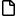DI Management Home > Mathematics > Python matrix tools over Zq

#Python matrix tools over $\mathbb{Z}_q$

This page provides the Python library matrixzq with functions to carry out matrix operations over $\mathbb{Z}_q$ ($\mathbb{Z}/q\mathbb{Z}$) in pure Python without using Numpy or Scipy.

Where q is a positive integer greater than 1. All matrix elements are expected to be nonnegative integers in the range [0, q-1]. All computations are carried out modulo q.

The modulus q does not have to be prime for most matrix arithmetical operations (add, multiply), but it must be prime to carry out operations that involve division (invert, solve).matrixzq.py Main library source codematrixzq_t.py Some testsmatrixzq-1.0.0.zip Download all as zip

## Background

We wanted pure Python code to operate on matrices modulo an integer q to demonstrate some principles of lattice-based encryption schemes using Learning-With-Errors (LWE). We found the excellent code "BASIC Linear Algebra Tools in Pure Python without Numpy or Scipy" by Thom Ives at

This code is inspired and partly derived from Thom's excellent work. Thank you, Thom.

We have provided basic Python functions rather than a more-complicated class so you can easily extract and work with the underlying list of lists.